# Cone A2V

Surface of cone in the plane is a circular arc with central angle of 126° and area 415 cm2.

Calculate the volume of a cone.

Result

V =  881.1 cm3

#### Solution:

$A=126 \cdot \ \dfrac{ \pi }{ 180 }=126 \cdot \ \dfrac{ 3.1416 }{ 180 } \doteq 2.1991 \ \text{rad} \ \\ S=415 \ \text{cm}^2 \ \\ \ \\ S=\pi s^2 \cdot \ A / (2 \pi) \ \\ \ \\ s=\sqrt{ 2 \cdot \ S/A }=\sqrt{ 2 \cdot \ 415/2.1991 } \doteq 19.4274 \ \text{cm} \ \\ r=A \cdot \ s/(2 \pi)=2.1991 \cdot \ 19.4274/(2 \cdot \ 3.1416) \doteq 6.7996 \ \text{cm} \ \\ h=\sqrt{ s^2-r^2 }=\sqrt{ 19.4274^2-6.7996^2 } \doteq 18.1986 \ \text{cm} \ \\ \ \\ V=\dfrac{ 1 }{ 3 } \cdot \ \pi \cdot \ r^2 \cdot \ h=\dfrac{ 1 }{ 3 } \cdot \ 3.1416 \cdot \ 6.7996^2 \cdot \ 18.1986 \doteq 881.1169 \doteq 881.1 \ \text{cm}^3$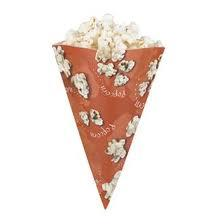Our examples were largely sent or created by pupils and students themselves. Therefore, we would be pleased if you could send us any errors you found, spelling mistakes, or rephasing the example. Thank you!

Leave us a comment of this math problem and its solution (i.e. if it is still somewhat unclear...):Be the first to comment!Tips to related online calculators
Need help calculate sum, simplify or multiply fractions? Try our fraction calculator.
Pythagorean theorem is the base for the right triangle calculator.

## Next similar math problems:

1. Storm and roof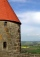The roof on the building is a cone with a height of 3 meters and a radius equal to half the height of the roof. How many m2 of roof need to be repaired if 20% were damaged in a storm?
2. Two bodiesThe rectangle with dimensions 8 cm and 4 cm is rotated 360º first around the longer side to form the first body. Then, we similarly rotate the rectangle around the shorter side b to form a second body. Determine the ratio of surfaces of the first and seco
3. Rectangular base pyramidCalculate an area of the shell of the pyramid with a rectangular base of 2.8 m and 1.4 m and height 2.5 meters.
4. Triangular prism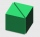Calculate the surface of a triangular prism with the base of an equilateral triangle with a side length of 7.5 cm and a corresponding height of 6.5 cm. Prism height is 15cm.
5. Calculate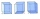Calculate the surface of a regular quadrilateral prism whose base edge is 2.4dm and the height of the prism is 38cm.
6. FlakesA circle was described on the square, and a semicircle above each side of the square was described. This created 4 "flakes". Which is bigger: the content of the central square or the content of four chips?
7. The basesThe bases of the isosceles trapezoid ABCD have lengths of 10 cm and 6 cm. Its arms form an angle α = 50˚ with a longer base. Calculate the circumference and content of the ABCD trapezoid.
8. Annulus from triangleCalculate the content of the area bounded by a circle circumscribed and a circle inscribed by a triangle with sides a = 25mm, b = 29mm, c = 36mm
9. A mapA map with a scale of 1: 5,000 shows a rectangular field with an area of 18 ha. The length of the field is three times its width. The area of the field on the map is 72 cm square. What is the actual length and width of the field?
10. Rectangular land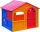On a rectangular land with dimensions of 35 m and 18.5 m is a house with a square floor plan with a side of 14 m. What % of the land is not occupied?
11. Compute 4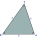Compute the exact value of the area of the triangle with sides 14 mi, 12 mi, and 12 mi long.
12. Playground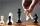On the special playground, there are 81 square sectors, each with a side of 5 m. How many players can fit on the playground if each player needs a 75 m2 area to play?
13. Two 2D shapes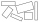Decide which shapes have more area:   (a) a square of 8cm side; or   (b) two rectangles with sides 5cm and 15cm? Write result as 1 or 2 (rectangles)
14. Garden exchangeThe garden has the shape of a rectangular trapezoid, the bases of which have dimensions of 60 m and 30 m and a vertical arm of 40 m. The owner exchanged this garden for a parallelogram, the area of which is 7/9 of the area of a trapezoidal garden. What is
15. Two gardens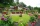The total area of the two gardens is 864 m2. The first garden is 60 m2 smaller than three times the second garden. What is the area of each garden?
16. NitrogenOne bag of urea containing 46 percent nitrogen weighs 25 kg. How many bags have to be purchased for fertilizing a field of 41003 square meters if the nitrogen dose is 50.0 kg per hectare?
17. Squares above sidesTwo squares are constructed on two sides of the ABC triangle. The square area above the BC side is 25 cm2. The height vc to the side AB is 3 cm long. The heel P of height vc divides the AB side in a 2: 1 ratio. The AC side is longer than the BC side. Calc